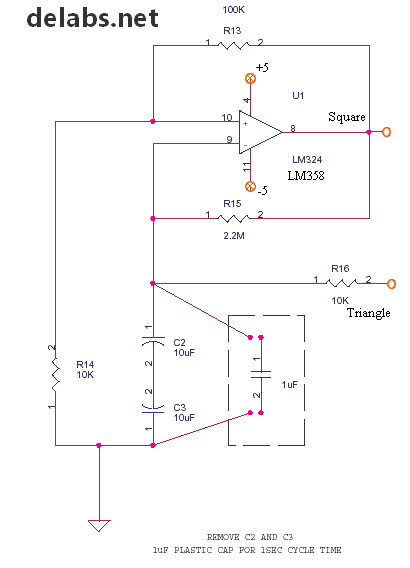## Schematics of delabs

One of the oldest Schematic Archives on the Web. It was in the form of pdf files in the late 90s. Instrumentation and Industrial Designs by delabs and Generic Application Designs.

## Sunday, March 20, 2016

### Square and Triangle Opamp Oscillator

Here is the circuit of a Op-Amp based Square Wave Generator. One of the main application of this is in a Simple PWM circuit and Triangle Opamp Oscillator. The Slope of the Triangle wave is compared with a DC Level to derive a pulse width or On-Time proportional to a Voltage.

Tutorials on Basics and Instrumentation Electronics

This On-Time proportional to voltage is for a fixed oscillation rate based on C2-C3 and R15. The C2-C3 form a Unpolarized cap, This type is also used in crossover networks in speaker boxes. This is because, a plastic 4.7 uF (2 * 10uF series) is big and costlier, but a plastic cap is very stable and closer to an ideal cap.First you need to know that most opamps in such circuits can give a square wave, but very few can swing to the rails (+/-5). For designing take 75% of Vcc. CA3130 is one that can swing to rails like a CMOS gate.

Assuming the swing of output is around +/- 4V, the pin 10 + input will be at +/- 2V. When the cap is discharged at 0 V, let us take that the output is +, the cap charges to a little above +2, - input becomes dominant, so output swings negative. This discharges the cap to 0 and then charges it negatively upto -2. This in turn flips the output to + as pin 10 turns dominant. Hence this continues as a oscillation, with a nice triangle across the cap for the PWM comparator.

Dominant means more +, 0 is positive compared to -2V. Also -2.5 is negative compared to -2.2.

Related Reading - Op Amp Triangle-Wave Generator